Courses

NCERT Solutions (Ex: 6.1 - 6.2) - Squares and Square Roots Notes - Class 8

Class 8: NCERT Solutions (Ex: 6.1 - 6.2) - Squares and Square Roots Notes - Class 8

The document NCERT Solutions (Ex: 6.1 - 6.2) - Squares and Square Roots Notes - Class 8 is a part of Class 8 category.
All you need of Class 8 at this link: Class 8

Exercise 6.1

Question 1:

What will be the unit digit of the squares of the following numbers?

(i) 81

(ii) 272

(iii) 799

(iv) 3853

(v) 1234

(vi) 26387

(vii) 52698

[viii] 99880

(ix) 12796

[x] 55555

(i) The number 81 contains its unit’s place digit 1. So, square of 1 is 1.

Hence, unit’s digit of square of 81 is 1.

(ii) The number 272 contains its unit’s place digit 2.

So, square of 2 is 4. Hence, unit’s digit of square of 272 is 4.

(iii) The number 799 contains its unit’s place digit 9.

So, square of 9 is 81. Hence, unit’s digit of square of 799 is 1.

(iv) The number 3853 contains its unit’s place digit 3.

So, square of 3 is 9. Hence, unit’s digit of square of 3853 is 9.

(v) The number 1234 contains its unit’s place digit 4.

So, square of 4 is 16. Hence, unit’s digit of square of 1234 is 6.

(vi) The number 26387 contains its unit’s place digit 7.

So, square of 7 is 49. Hence, unit’s digit of square of 26387 is 9.

(vii) The number 52698 contains its unit’s place digit 8.

So, square of 8 is 64. Hence, unit’s digit of square of 52698 is 4.

(viii) The number 99880 contains its unit’s place digit 0.

So, square of 0 is 0. Hence, unit’s digit of square of 99880 is 0.

(ix) The number 12796 contains its unit’s place digit 6.

So, square of 6 is 36. Hence, unit’s digit of square of 12796 is 6.

(x) The number 55555 contains its unit’s place digit 5.

So, square of 5 is 25. Hence, unit’s digit of square of 55555 is 5.

Question 2:

The following numbers are obviously not perfect squares. Give reasons.

[i] 1057

[ii] 23453

(iii] 7928

(iv] 222222

[v] 64000

(vi) 89722

(vii) 222000

(viii) 505050

(i) Since, perfect square numbers contain their unit’s place digit 1, 4, 5, 6, 9 and even numbers of 0.

Therefore 1057 is not a perfect square because its unit’s place digit is 7.

(ii) Since, perfect square numbers contain their unit’s place digit 0, 1, 4, 5, 6, 9 and even number of 0.

Therefore 23453 is not a perfect square because its unit’s place digit is 3.

(iii) Since, perfect square numbers contain their unit’s place digit 0, 1, 4, 5, 6, 9 and even number of 0.

Therefore 7928 is not a perfect square because its unit’s place digit is 8.

(iv) Since, perfect square numbers contain their unit’s place digit 0, 1, 4, 5, 6, 9 and even number of 0.

Therefore 222222 is not a perfect square because its unit’s place digit is 2.

(v) Since, perfect square numbers contain their unit’s place digit 0, 1, 4, 5, 6, 9 and even number of 0.

Therefore 64000 is not a perfect square because its unit’s place digit is single 0.

(vi) Since, perfect square numbers contain their unit’s place digit 0, 1, 4, 5, 6, 9 and even number of 0.

Therefore 89722 is not a perfect square because its unit’s place digit is 2.

(vii) Since, perfect square numbers contain their unit’s place digit 0, 1, 4, 5, 6, 9 and even number of 0.

Therefore 222000 is not a perfect square because its unit’s place digit is triple 0.

(viii) Since, perfect square numbers contain their unit’s place digit 0, 1, 4, 5, 6, 9 and even number of 0.

Therefore 505050 is not a perfect square because its unit’s place digit is 0.

Question 3:

The squares of which of the following would be odd number:

(i) 431

(ii) 2826

(iii) 7779

(iv) 82004

Answer 3: (i) 431 – Unit’s digit of given number is 1 and square of 1 is 1.

Therefore, square of 431 would be an odd number.

(ii) 2826 – Unit’s digit of given number is 6 and square of 6 is 36.

Therefore, square of 2826 would not be an odd number.

(iii) 7779 – Unit’s digit of given number is 9 and square of 9 is 81.

Therefore, square of 7779 would be an odd number.

(iv) 82004 – Unit’s digit of given number is 4 and square of 4 is 16.

Therefore, square of 82004 would not be an odd number.

Question 4:

Observe the following pattern and find the missing digits: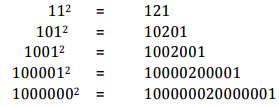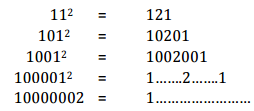Question 5:

Observe the following pattern and supply the missing numbers: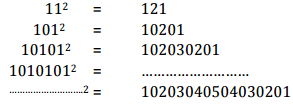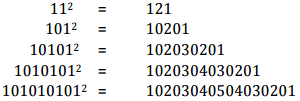Question 6:

Using the given pattern, find the missing numbers: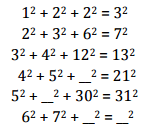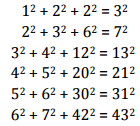Question 7:

(i) 1 + 3 + 5 + 7 + 9

(ii) 1 + 3 + 5 + 7 + 9 + 11 + 13 + 15 + 17 + 19

(iii) 1 + 3 + 5 + 7 + 9 + 11 + 13 + 15 + 17 + 19 + 21 + 23

[i]Here,there are five odd numbers. Therefore square of 5 is 25.

∴ 1 + 3 + 5 + 7 + 9 = 52 = 25

[ii] Here, there are ten odd numbers. Therefore square of 10 is 100.

∴ 1 + 3 + 5 + 7 + 9 + 11 + 13 + 15 + 17 + 19 = 102 = 100

[iii]

Here, there are twelve odd numbers. Therefore square of 12 is 144.

∴ 1 + 3 + 5 + 7 + 9 + 11 + 13 + 15 + 17 + 19 + 21 + 23 = 122 = 144

Question 8:

(i) Express 49 as the sum of 7 odd numbers.

(ii) Express 121 as the sum of 11 odd numbers.

(i) 49 is the square of 7. Therefore it is the sum of 7 odd numbers.

49 = 1 + 3 + 5 + 7 + 9 + 11 + 13

(ii) 121 is the square of 11. Therefore it is the sum of 11 odd numbers

121 = 1 + 3 + 5 + 7 + 9 + 11 + 13 + 15 + 17 + 19 + 21

Question 9:

How many numbers lie between squares of the following numbers:

(i) 12 and 13

(ii) 25 and 26

(iii) 99 and 100

(i)

Since, non-perfect square numbers between n2 and (n + 1)"are  2n

Here, n = 12

Therefore, non-perfect square numbers between 12 and 13 = 2 n = 2x12 = 24

[ii]

Since, non-perfect square numbers between n2 and (n + 1)2 are 2n.

Here, n - 25

Therefore, non-per feet square numbers between 25 and 26 = 2 n = 2x25 = 50

[iii]

Since, non-perfect square numbers between n and (n + 1) are 2n.

Here, n = 99

Therefore, non-perfect square numbers between 99 and 100 = 2n = 2 x 99 = 198

Exercise 6.2

Question 1: Find the squares of the following numbers:

(i) 32

(ii) 35

(iii) 86

(iv) 93

(v) 71

(vi) 46

(i) (32)= (30 + 2)2 = (30)2 + 2x 30x 2 + (2)2  [∵ (a+b)2 = a2 + b2 + 2ab]

= 900+ 120 + 4 = 1024

(ii)

(35)2 = (30 + 5)= (30)2 + 2x 30x 5 + (5)2  [∵ (a+b)2 = a2 + b2 + 2ab]

= 900 + 300 + 25 = 1225

(iii)

(86)= (80 + 6)2 = (80)2+ 2 x 80 x 6 + (6)2     [∵ (a+b)2 = a2 + b2 + 2ab]

= 1600 + 960 + 36 = 7386

(iv)

(93)2 =(90 +3)2 =(90)2 +2x90x3 + (3)    [∵ (a+b)2 = a2 + b2 + 2ab]

= 8100 + 540 + 9 = 8649

(v)

(71)2 = (70+1)2 = 70 + 2 x 70 x 1 + 12 [∵ (a+b)2 = a2 + b2 + 2ab]

= 4900 + 140 + 1 = 5041

[vi]

(46)2 =(40 +6)2 = (40)2+2x40x6 +(6)2   [∵ (a+b)2 = a2 + b2 + 2ab]

= 1600 + 480 + 36 = 2116

Question 2:

Write a Pythagoras triplet whose one member is:

(i) 6

(ii) 14

(iii) 16

(iv) 18

(i)

There are three numbers 2m,m 2 -1 and m2 + 1 in a Pythagorean Triplet

Here, 2m 6  =>  m = 6/2 = 3

There fore,

Second number (m2 -1) = (3)2- 1 9 -1 8

Third number m2+1 (3)2 +1 = 9 + 1 = 10

Hence, Pythagorean triplet is (6,8,10).

(ii)

There are three numbers 2m, m-1 and nr +1 in a Pythagorean Triplet

Here, 2m = 6, m = 6/2 = 3

Therefore,

Second number (m2 - 1) = (7)2 -1 = 49 - 1 = 48

Third number m2 +1 = (7)2 + l =49 + l = 50

Hence, Pythagorean triplet is (14,48, 50).

(iii)

There are three numbers 2m,m2 -1 and m2 + 1 in a Pythagorean Triplet

Here, 2m =16  , m = 8

Therefore,

Second number (m2 - 1) = (8)2 -1 =64- 1 = 63

Third number m2 +1 = (8)2 +1 = 64 +1 = 65

Hence, Pythagorean triplet is (16, 63, 65).

(iv)

There are three numbers 2m,m2 -1 and m2 + 1 in a Pythagorean Triplet

Here, 2m =18 , m = 9

Therefore,

Second number (m2 - 1) = (9)2 -1 = 81- 1 = 80

Third number m2 +1 = (9)2 +1 = 81 +1 = 62

Hence, Pythagorean triplet is (18, 80, 82).

The document NCERT Solutions (Ex: 6.1 - 6.2) - Squares and Square Roots Notes - Class 8 is a part of Class 8 category.
All you need of Class 8 at this link: Class 8Use Code STAYHOME200 and get INR 200 additional OFF Use Coupon Code

Top Courses for Class 8Top Courses for Class 8Track your progress, build streaks, highlight & save important lessons and more!

,

,

,

,

,

,

,

,

,

,

,

,

,

,

,

,

,

,

,

,

,

;